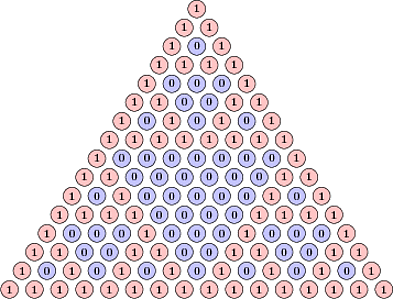## Playing with Pascal’s Triangle

The beautiful Pascal triangle has been around for many years. Can you say something new about it?Of course you can. Mathematicians always find new way to look at things. In 2012 RSI student, Kevin Garbe, did some new and cool research related to the triangle. Consider Pascal’s triangle modulo 2, see picture which was copied from a stackexchange discussion.

A consecutive block of m digits in one row of the triangle modulo 2 is called an m-block. If you search the triangle you will find that all possible binary strings of length 2 are m-blocks. Will this trend continue? Yes, you can find any possible string of length 3, but it stops there. The blocks you can find are called accessible blocks. So, which blocks of length 4 are not accessible?

There are only two strings that are not accessible: 1101 and 1011. It is not surprising that they are reflections of each other. Pascal’s triangle respects mirror symmetry and the answer should be symmetric with respect to reflection.

You can’t find these blocks on the picture, but how do we prove that they are not accessible, that is, that you can’t ever find them? The following amazing property of the triangle can help. We call a row odd/even, if it corresponds to binomial coefficients of n choose something, where n is an odd/even number. Every odd row has every digit doubled. Moreover, if we take odd rows and replace every double digit with its single self we get back Pascal’s triangle. Obviously the two strings 1101 and 1011 can’t be parts of odd rows.

What about even rows? The even rows have a similar property: every even-indexed digit is a zero. If you remove these zeros you get back Pascal’s triangle. The two strings 1101 and 1011 can’t be part of even rows. Therefore, they are not accessible.

The next question is to count the number of inaccessible blocks of a given length: a(n). This and much more was done by Kevin Garbe for his RSI 2012 project. (I was the head mentor of the math projects.) His paper is published on the arxiv. The answer to the question can be found by constructing recurrence relations for odd/even rows. It can be shown that a(2r) = 3a(r) + a(r+1) − 6 and a(2r+1) = 3a(r) + 2a(r+1) − 6. As a result the number of inaccessible blocks of length n is n2n + 2. I wonder if there exists a direct proof of this formula without considering odd and even rows separately.

This RSI result was so pretty that it became a question at our entrance PRIMES test for the year 2013. In the test we changed the word accessible to admissible, so that it would be more difficult for applicants to find the research. Besides, Garbe’s paper wasn’t arxived yet.

The pretty picture above is from the stackexchange, where one of our PRIMES applicants tried to solicit help in solving the test question. What a shame.

Share:

### One Comment

1. #### Ko-Wei Lih:

Please see the solutions to problem E2775 on pp. 578-580 of the American Mathematical Monthly, Vol. 87, No. 7 (Aug.-Sep., 1980).